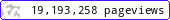•••••MadAsMaths.com :: Project Maths :: Starters :: Data Handling

PROJECT MATHS - STARTERS - DATA HANDLING

These presentations contain short activities, often suitable for revision, on a variety of mathematical topics of statistical nature, including probability.

Note that this is a licensed product if it is to be used for “classroom teaching” so schools or academic institutions must first purchase a licence. Click HERE to contact me.

 averages_a_mean_starter_starter.pptaverages_and_range_starter.pptbar_chart_proportion_starter.pptcorrelation_starter.pptcum_freq_graph_starter.ppthalfway_mean_starter.pptmean_and_range_starter.pptmean_by_coding_starter.pptpie_chart_bar_chart_starter.pptpie_chart_match_with_data_starter.pptpie_chart_percentages_starter.pptpie_chart_proportion_starter.pptpie_chart_starter.pptpie_charts_proportionality_calculations_starter.pptprobability_bag_starter.pptprobability_balls_starter.pptprobability_card_calculation_starter.pptprobability_cards_starter.pptprobability_classify_numbers_starter.pptprobability_dice_starter.pptprobability_scale_starter.pptprobability_spinner_practice_starter.pptprobability_spinner_starter.pptprobability_worded_starter.pptsurvey_and_display_starter.ppt